International
Tables for
Crystallography
Volume A
Space-group symmetry
Edited by M. I. Aroyo

International Tables for Crystallography (2016). Vol. A, ch. 1.2, pp. 12-13

Section 1.2.1. Crystallographic symmetry operations

H. Wondratscheka and M. I. Aroyob

aLaboratorium für Applikationen der Synchrotronstrahlung (LAS), Universität Karlsruhe, Germany, and bDepartamento de Física de la Materia Condensada, Universidad del País Vasco (UPV/EHU), Bilbao, Spain

1.2.1. Crystallographic symmetry operations

| top | pdf |

Geometric mappings have the property that for each point P of the space, and thus of the object, there is a uniquely determined point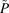, the image point. If also for each image pointthere is a uniquely determined preimage or original point P, then the mapping is called reversible. Non-reversible mappings are called projections, cf. Section 1.4.5.

A mapping is called a motion, a rigid motion or an isometry if it leaves all distances invariant (and thus all angles, as well as the size and shape of an object). In this volume the term isometry' is used.

 Isometries are a special kind of affine mappings. In an affine mapping, parallel lines are mapped onto parallel lines; lengths and angles may be distorted but distances along the same line are preserved. A mapping is called a symmetry operation of an object if (i) it is an isometry, and (ii) it maps the object onto itself. Instead of maps the object onto itself' one frequently says `leaves the object invariant (as a whole)'. Real crystals are finite objects in physical space, which because of the presence of impurities and structural imperfections such as disorder, dislocations etc. are not perfectly symmetric. In order to describe their symmetry properties, real crystals are modelled as blocks of ideal, infinitely extended periodic structures, known as ideal crystals or (ideal) crystal structures. Crystal patterns are models of crystal structures in point space. In other words, while the crystal structure is an infinite periodic spatial arrangement of the atoms (ions, molecules) of which the real crystal is composed, the crystal pattern is the related model of the ideal crystal (crystal structure) consisting of a strictly three-dimensional periodic set of points in point space. If the growth of the ideal crystal is undisturbed, then it forms an ideal macroscopic crystal and displays its ideal shape with planar faces. Both the symmetry operations of an ideal crystal and of a crystal pattern are called crystallographic symmetry operations. The symmetry operations of the ideal macroscopic crystal form the finite point group of the crystal, those of the crystal pattern form the (infinite) space group of the crystal pattern. Because of its periodicity, a crystal pattern always has translations among its symmetry operations. The symmetry operations are divided into two main kinds depending whether they preserve or not the so-called handedness or chirality of chiral objects. Isometries of the first kind or proper isometries are those that preserve the handedness of chiral objects: e.g. if a right (left) glove is mapped by one of these isometries, then the image is also a right (left) glove of equal size and shape. Isometries that change the handedness, i.e. the image of a right glove is a left one, of a left glove is a right one, are called isometries of the second kind or improper isometries. Improper isometries cannot be performed in space physically but can nevertheless be observed as symmetries of objects. The notion of fixed points is essential for the characterization of symmetry operations. A point P is a fixed point of a mapping if it is mapped onto itself, i.e. the image pointis the same as the original point P: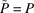. The set of all fixed points of an isometry may be the whole space, a plane in the space, a straight line, a point, or the set may be empty (no fixed point). Crystallographic symmetry operations are also characterized by their order: a symmetry operation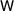is of order k if its application k times results in the identity mapping, i.e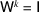, whereis the identity operation, and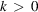is the smallest number for which this equation is fulfilled.

There are eight different types of isometries that may be crystallographic symmetry operations:

 (1) The identity operationmaps each point of the space onto itself, i.e. the set of fixed points is the whole space. It is the only operation whose order is 1. The identity operation is a symmetry operation of the first kind. It is a symmetry operation of any object and although trivial, it is indispensable for the group properties of the set of symmetry operations of the object (cf. Section 1.1.2). (2) A translationis characterized by its translation vector t. Under translation every point of space is shifted by t, hence a translation has no fixed point. A translation is a symmetry operation of infinite order as there is no number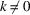such that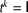with translation vector o. It preserves the handedness of any chiral object. (3) A rotation is an isometry which leaves one line fixed pointwise. This line is called the rotation axis. The degree of rotation about this axis is described by its rotation angle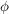. Because of the periodicity of crystals, the rotation angles of crystallographic rotations are restricted to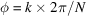, where N = 2, 3, 4 or 6 and k is an integer which is relative prime to N. A rotation of rotation angleis of order N and is called an N-fold rotation. A rotation preserves the handedness of any chiral object. The rotations are also characterized by their sense of rotation. The adopted convention for positive (negative) sense of rotation follows the mathematical convention for positive (negative) sense of rotation: the sense of rotation is positive (negative) if the rotation is counter-clockwise (clockwise) when viewed down the rotation axis. (4) A screw rotation is a rotation coupled with a translation parallel to the rotation axis. The rotation axis is called the screw axis. The translation vector is called the screw vector or the intrinsic translation component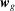(of the screw rotation), cf. Section 1.2.2.4. A screw rotation has no fixed points because of its translation component. However, the screw axis is invariant pointwise under the so-called reduced symmetry operation of the screw rotation: it is the rotation obtained from the screw rotation by removing its intrinsic translation component. The screw rotation is a proper symmetry operation. If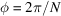is the smallest rotation angle of a screw rotation, then the screw rotation is called N-fold. Owing to its translation component, the order of any screw rotation is infinite. Let u be the shortest lattice vector in the direction of the screw axis, and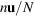, with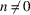and integer, be the screw vector of the screw rotation by the angle. After N screw rotations with rotation anglethe crystal pattern has its original orientation but is shifted parallel to the screw axis by the lattice vector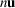. (5) An N-fold rotoinversion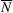is an N-fold rotation coupled with an inversion through a point on the rotation axis. This point is called the centre of the rotoinversion. For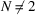it is the only fixed point. The axis of the rotation is invariant as a whole under the rotoinversion and is called its rotoinversion axis. The restrictions on the anglesof the rotational parts are the same as for rotations. The order of an N-fold rotoinversion is N for even N and 2N for odd N. A rotoinversion changes the handedness by its inversion component: it maps any right-hand glove onto a left-hand one and vice versa. Special rotoinversions are those for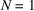and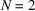which are dealt with separately. The rotoinversionscan be described equally as roto­reflections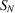. The N-fold rotation is now coupled with a reflection through a plane which is perpendicular to the rotation axis and cuts the axis in its centre. The following equivalences hold: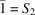,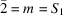,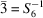,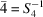and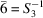. In this volume the description by rotoinversions is chosen. (6) The inversion can be considered as a onefold rotoinversion (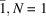) or equally as a twofold rotoreflection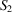. The fixed point is called the inversion centre. The inversion is a symmetry operation of the second kind, its order is 2. (7) A twofold rotoinversion () is equivalent to a reflection or a reflection through a plane and is simultaneously a onefold rotoreflection (). It is an isometry which leaves the plane perpendicular to the twofold rotoinversion axis fixed pointwise. This plane is called the reflection plane or mirror plane; it intersects the rotation axis in its centre. Its orientation is described by the direction of its normal vector, i.e. of the rotation axis. (Note that in the space-group tables of Part 2 the reflection planes are specified by their locations, and not by their normal vectors, cf. Section 1.4.2.1.) The order of a reflection is 2. As for any rotoinversion, the reflection changes the handedness of a chiral object. (8) A glide reflection is a reflection through a plane coupled with a translation parallel to this plane. The translation vector is called the glide vector (or the intrinsic translation componentof the glide reflection, cf. Section 1.2.2.4). A glide reflection changes the handedness and has no fixed point. The set of fixed points of the related reduced symmetry operation (i.e. the reflection that is obtained by removing the glide component from the glide reflection) is called the glide plane. The glide vector of a glide reflection is 1/2 of a lattice vector t (including centring translations of centred-cell lattice descriptions, cf. Table 2.1.1.2). Whereas twice the application of a reflection restores the original position of the crystal pattern, applying a glide reflection twice results in a translation of the crystal pattern with the translation vector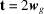. The order of any glide reflection is infinite.